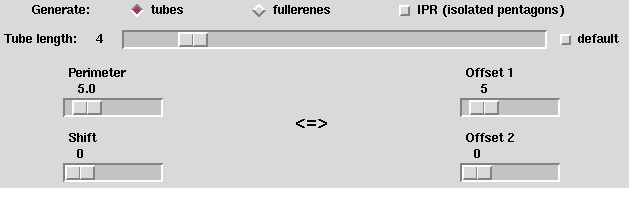hello Up : Main Prev : Generation programs Next : HCgen

CaGe V0.3

Tubetype

Tubetype is a generation program which generates tubes or fullerenes that can be split into two tubes. Such fullerenes are called tube--type fullerenes. Like fullerenes, tubes consist of only pentagons (exactly 6 pentagons) and hexagons. However, only inner vertices are adjacent to three other vertices. Tubes have an outer cycle where the vertices are alternately 2- and 3--valent, with two exceptions: There is one place on the outer cycle where two successive vertices are 2--valent and one place where two successive vertices are 3--valent. There are two different ways to measure the distance between these places (the following description is not precise, it gives only a clue):• Perimeter and shift: The perimeter is the length of the outer cycle. It is measured in hexagon units. The shift is the number of 3--valent vertices between the two places.
• Offsets: The length of the outer cycle is given implicitly by giving two offsets which denote the number of 3--valent vertices between the two places. Two values are necessary because there are two paths on the cycle which connect the two places.
• Basic options: By the radiobuttons named "tubes" and "fullerenes" you determine what kind of objects to be generated. If the "IPR"--checkbutton is switched on, then only objects with isolated pentagons are generated. When a tube is generated, it can easily be extended to a tube with more vertices by adding hexagons to the outer cycle. One complete ring consisting of hexagons must be added. In this case the resulting outer cycle after adding the ring looks the same as before. So each tube has a number of hexagon rings called the tube length. The tube length can be chosen by the "Tube length"--slider. If the "default"--checkbutton is switched on, then the default tube length will be taken which depends on the perimeter and shift. For fullerenes, the tube length means the number of inner hexagon rings. The "Perimeter", "Shift", "Offset 1" and "Offset 2"--sliders are used to choose the related parameters as described above. You should use either "Perimeter" and "Shift", or "Offset 1" and "Offset 2", depending on the kind of imagination you prefer. There are several interactions between the sliders. Note that not all values for "Offset 1" can be translated to "Perimeter" values since then the limit for "Perimeter" would have to be raised.
Output options: See section fullgen .